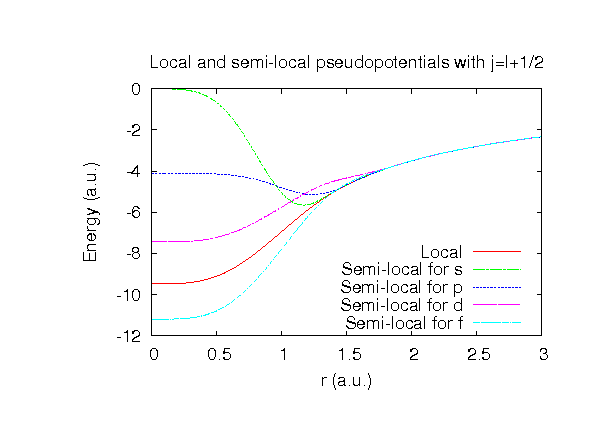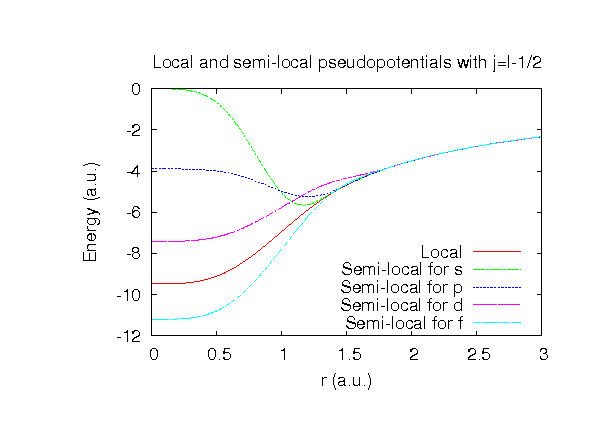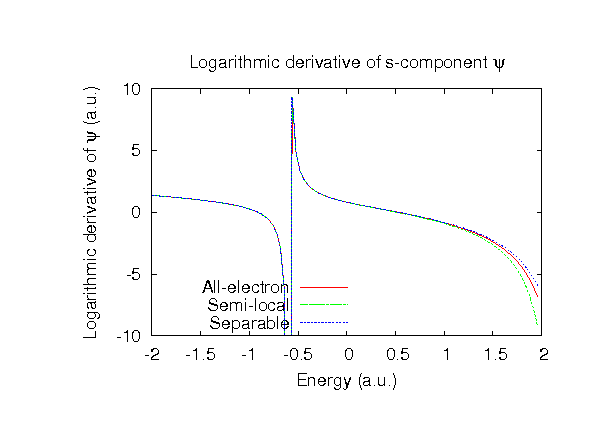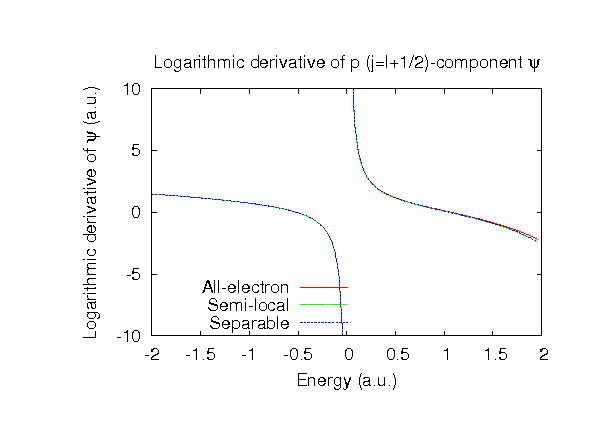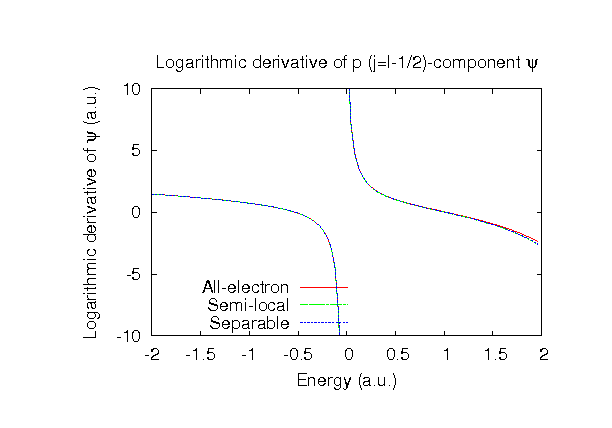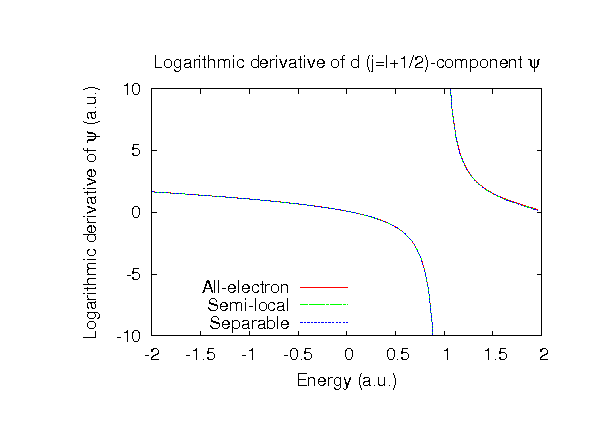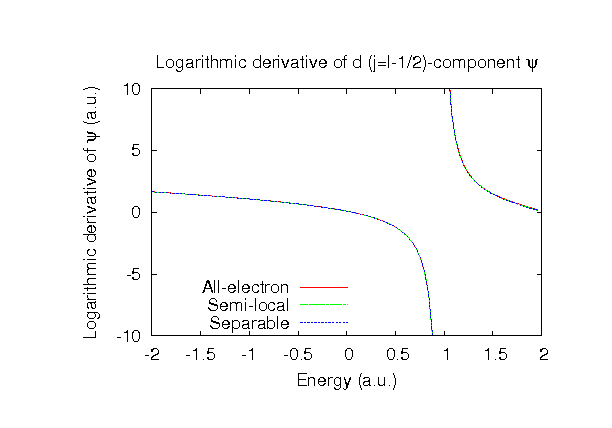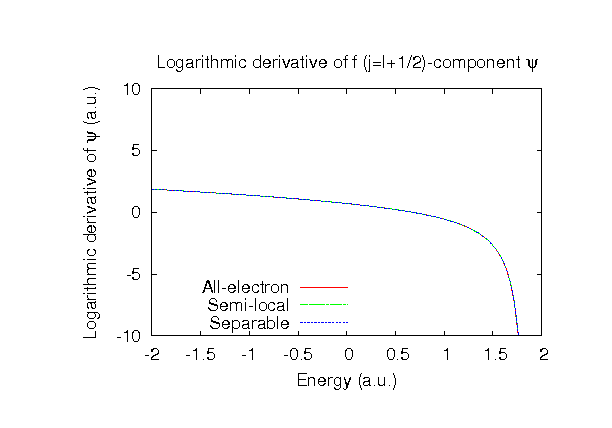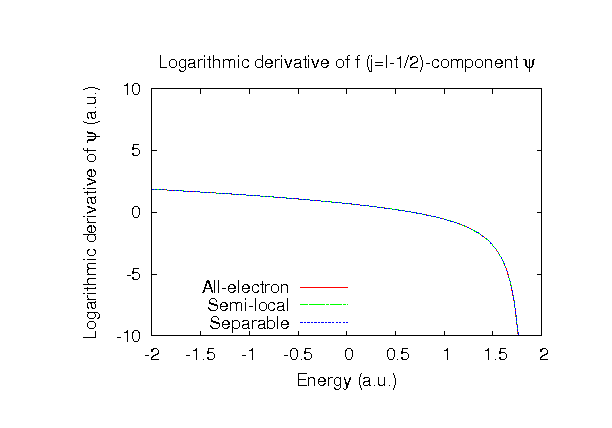### Fully relativistic pseudopotentials

Fully relativistic pseudopotentials generated by the MBK (PRB 47, 6728 (1993)) scheme within LDA (CA13) and GGA (PBE13) which contain a partial core correction and fully relativistic effects including spin-orbit coupling.

### Pseudo-atomic orbitals

The number below the symbol means a cutoff radius (a.u.) of the confinement potential. These file includes fifteen radial parts for each angular momentum quantum number l (=0,1,2,3,4). The basis functions were generated by variationally optimizing the corresponding primitive basis functions in the single atom, the dimer molecule, and the NaBr bulk. The input files used for the orbital optimization can be found at Br_opt.dat , Br2_opt.dat , and NaBr_opt.dat . Since Br_CA13.vps and Br_PBE13.vps include the 4s and 4p (7 electrons) as the valence states, the minimal basis set is Br*.*-s1p1. Our recommendation for the choice of cutoff radius of basis functions is that Br7.0.pao is enough for bulks, but Br8.0.pao or Br9.0.pao is preferable for molecular systems.

### Benchmark calculations by the PBE13 pseudopotential with the various basis functions

(1) Calculations of the total energy of NaBr in the rock salt structure as a function of lattice constant, where the total energy is plotted relative to the minimum energy for each case. a0 and B0 are the equilibrium lattice constant and bulk modulus obtained by fitting to the Murnaghan equation of state. The difference between Br7.0-s3p2d1 and Br7.0-s3p3d2 in the total energy at the minimum point is 0.0076 eV/atom, where Na9.0-s3p2d1 was used as basis functions for Na for both the cases. An input file used for the OpenMX calculations can be found at NaBr-EvsV.dat . For comparison the result by the Wien2k code is also shown, where the calculation was performed by default setting in the Ver. 9.1 of Wien2k except for the use of RMT x KMAX of 12.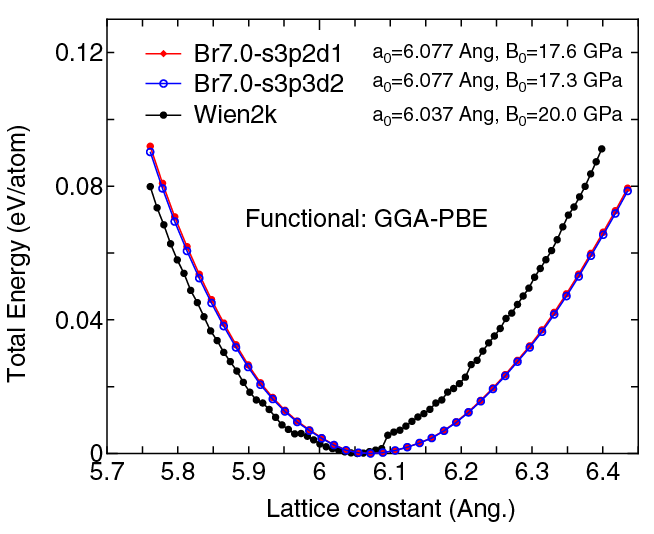(2) Calculations of the band dispersion of NaBr in the rock salt structure, where the non-spin polarized collinear calculation with the lattice constant of 5.961 Ang. was performed using Na_PBE.vps, Br_PBE13.vps, Na9.0-s3p2d1, and Br7.0-s3p2d1, and the origin of the energy is taken to be the top of the valence band. The input file used for the OpenMX calculations can be found at NaBr-Band.dat . For comparison the result by the Wien2k code is also shown, where the calculation was performed by default setting in the Ver. 9.1 of Wien2k except for the use of RMT x KMAX of 12.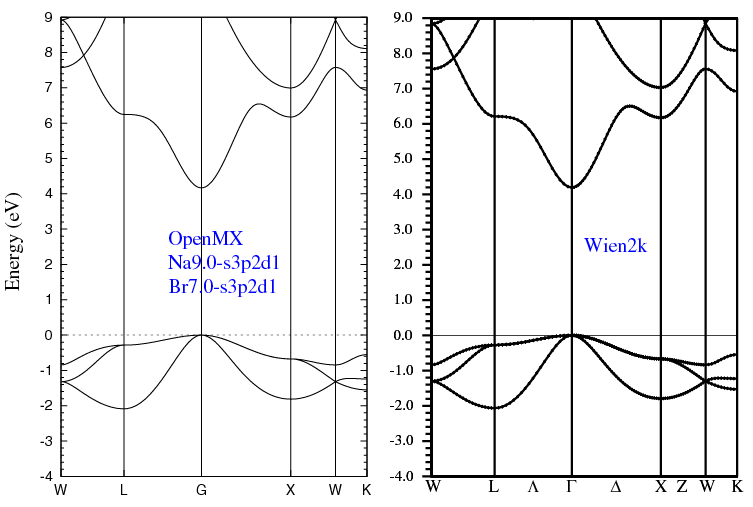(3) Calculations of the Br2 molecule with S=1, where Br_PBE13.vps and Br9.0-s3p3d2f1 or Br9.0-s4p4d4f1 were used. The input files used for the OpenMX calculations can be found at Br2.dat , Br.dat , and Br_CP.dat .

 Equilibrium bond length (Ang.) Atomization energy (eV) Atomization energy (couterpoise corrected) (eV) Br9.0-s3p3d2f1 2.340 2.438 2.436 Br9.0-s4p4d3f1 2.324 2.510 2.509 Other calc. 2.309 a 2.495 a Expt. 2.281 b 2.295 b

a W.A. Al-Saidi, J. Chem. Phys. 129, 064316 (2008).
b Taken from Ref. (a)

### Supplementary information for the GGA (PBE13) pseudopotential